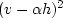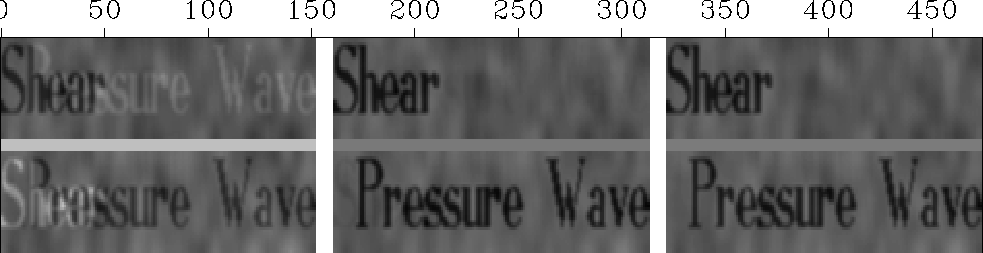Next: Clarity of nonlinear picture Up: Solution by weighting functions Previous: Solution by weighting functions

### A nonlinear-estimation method

What I have described above represents my first iteration. It can be called a linear-estimation method." Next we will try a nonlinear-estimation method" and see that it works better. If we think of minimizing the relative error in the residual, then in linear estimation we used the wrong divisor--that is, we used the squared data v2 where we should have used the squared residual.Using the wrong divisor is roughly justified when the crosstalkis small because then v2 andare about the same. Also, at the outset the residual was unknown, so we had no apparent alternative to v2, at least until we found.Having found the residual, we can now use it in a second iteration. A second iteration causesto change a bit, so we can try again. I found that, using the same data as in Figure 1, the sequence of iterations converged in about two iterations.reswait
Figure 2
Comparison of weighting methods. Left shows crosstalk as badly removed by uniformly weighted least squares. Middle shows crosstalk removed by deriving a weighting function from the input data. Right shows crosstalk removed by deriving a weighting function from the fitting residual. Press button for movie over iterations.

Figure 2 shows the results of the various weighting methods. Mathematical equations summarizing the bottom row of this figure are:(9) (10) (11)
For the top row of the figure, these equations also apply, butandshould be swapped.Next: Clarity of nonlinear picture Up: Solution by weighting functions Previous: Solution by weighting functions
Stanford Exploration Project
10/21/1998